Study Guide - Part 2 Theory Which Invalidates Itself
 Introductioninstein's "theory" of relativity is invalid in its entirety because of reasons inherent in it. It is destroyed at once by the very formulae which Einstein derives in the stationary system K when discussing the thought experimenthe has presented in §2 of , pivotal for that theory. These formulae are naively aimed at proving the crucial claim, which the entire theory  is based on, namely, that the same two clocks which are synchronous for the observers at rest with these clocks in the stationary system K are non-synchronous (which according to the theory is a judgement of the observers in K) for the observers at rest with these same clocks in the moving system k. That claim Einstein has clothed in words in the following general way: "So we see that we cannot attach any absolute signification to the concept of simultaneity, but that two events which, viewed from a system of co-ordinates, are simultaneous, can no longer be looked upon as simultaneous events when envisaged from a system which is in motion relatively to that system." The above claim, later called "relativity of simultaneity" by Einstein, which is the very heart of the theory, is untenable. This causes the collapse of Einstein's theory of relativity altogether. There is nothing in Einstein's theory of relativity, once "relativity of simultaneity" is proven invalid, which would remain or would be of any use for physics or science in general. Prerequisites To understand the failure to prove "relativity of simultaneity" it should first be clear that the claim mentioned in  is based solely on the derivations carried out by the observers in the stationary system K. These observers have at their disposal only the parameters in their own system K. Therefore, the conclusions, both in what we denote here as the first part of the claim, referring to the stationary system K, and in the second part of the claim, referring to the moving system k, stem only from these parameters in K. Further, to aid in achieving the goal of the theory in , namely, to enable the observers in K make a conclusion as to what the observers in k see, which the conclusion-making observers in K have no way to know directly, Einstein assumes and expresses explicitly the obvious fact that spatially coinciding clocks are synchronous in the stationary system K: "We imagine further that at the two ends A and B of the rod, clocks are placed which synchronize with the clocks of the stationary system, that is to say that their indications correspond at any instant to the "time of the stationary system'' at the places where they happen to be. These clocks are therefore "synchronous in the stationary system"." In this way the observers in the stationary system K can conveniently tell what the readings are of any clock which happens to coincide spatially with a clock of known reading in their system K since these coinciding clocks will show the same time equal to the known time. Theory Once the above is understood well, one is ready to proceed with the analysis, which reveals conclusively that the attempt to prove the claim for "relativity of simultaneity"is a failed attempt. In ruminating about the failure of the mentioned claim one should not be mislead by the fact that what we denoted here as its first part, stating that "observers in the stationary system would declare the clocks to be synchronous" is certainly confirmed by the mentioned formulae for completeness shown below in a general form), derived in K -- it is seen that the clocks positioned in the stationary system K, where the ends A and B of the moving rod happen to be in K, are synchronous; the formulae show that both clocks in K read the same time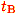on their faces which means, in agreement with the above-quoted first part of the claim, that these clocks are synchronous in K: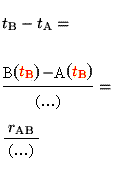andwhere (…) denotes either,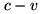or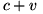. Disappointingly, however, the second part of the claim, which, recall, is a claim by the conclusion-making observers in K, stating that "Observers moving with the moving rod would thus find that the two clocks were not synchronous" is incorrect because the clocks discussed in the quote, moving with the moving rod (the clocks in the moving system k, that is), coincide spatially with the same clocks in K which, as just discussed, the above formulae show are synchronous in K, both showing timeon their faces. Therefore, the clocks placed at end A and at end B of the rod, moving with the moving rod, also both show timeon their faces, that is, they are synchronous. Therefore, contrary to the statement in the above quote, observers moving with the moving rod would thus necessarily find that the two clocks were synchronous. In summary, the truth is that two synchronous clocks in K remain synchronous also in k which means that, contrary to Einstein's impression, simultaneity is absolute. As seen, this truth is confirmed by the formulae given by Einstein in §2 of , even if they are presented in most general form, as is done above, independent of whether or not the second postulate is considered as valid or independent of whether or not the source of light is at rest with respect to the stationary system (that is, is immovably attached to the moving rod). Thus, purportedly one of the greatest discoveries of all time called "relativity of simultaneity" turns out to be no discovery at all but a most trivial mistake due to incorrect analysis of elementary formulae from Newton's mechanics. As seen, the truth these formulae contain, which can be revealed when said formulae are properly analyzed, is that simultaneity is absolute. The conclusion contradicting absoluteness of simultaneity, that is, the conclusion for the "relativity of simultaneity", is arrived at only if the mentioned truth following from the mentioned initial condition, namely, that the valid mechanics in K is the Newton's mechanics, is deliberately "forgotten" and the analysis is based solely on another initial condition, postulating that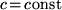is the universal velocity of light. Thus, by postulating an initial condition such as the ubiquitousEinstein reaches a conclusion in contradiction with a conclusion following from another, inevitable at that, initial condition, namely, the obvious validity of Newton's mechanics in K. Clearly, it is utterly unacceptable to postulate anything that would lead to a contradiction with elementary truths about a system of reference. The truth cannot be overruled by deciding to postulate something which would lead to overruling that truth. Summary The above concludes the analysis -- Einstein's theory of relativity has been definitively proven to be invalid in its entirety due to reasons internal to it. Thus, any further discussion of that theory is superfluous and will bring nothing new to the above categorical proof for its invalidity. Any further considerations of that theory would be reasonable for educational purposes only and/or for examining the ramifications of its invalidity -- the invalidity of Einstein's theory of relativity also causes any other theory or theories such as string theories, cosmology etc. which are based on it to be invalid. The above also proves that Einstein's theory of relativity could have never possibly been nor can ever be shown experimentally or otherwise to be valid. All claims to the opposite are either due to undetected errors or are due to unscrupulous manipulation of the public opinion. Further Educational Exercises In the spirit of exercise one may further want to analyze the above-mentioned internal contradiction between the two initial conditions, one inevitable (Newton's mechanics valid in K), the other postulated (velocity of light"independent of the state of motion of the emitting body". That internal contradiction expresses itself further in obviously unacceptable contradictiory statements about the same objects in one and the same system. Those contradictory statements, following from Einstein's theory of relativity, about the same objects in the same system, can serve as yet another, although redundant, proof for the invalidity of said theory. The culprit in question leading to the discussed internal contradiction, namely, the postulated velocity of light"independent of the state of motion of the emitting body", has enabled Einstein to carry out his manipulation, as said, by seemingly forgetting the fact shown above that his very formulae prove persistent synchronicity in all systems of two clocks synchronous in a given system. Recall, this directly follows from the fact explicitly acknowledged as well by Einstein himself  that the valid mechanics in the stationary system K where the derivations are carried out is the Newtonian mechanics. Before giving links to arguments regarding the culprit in question, notice a more than telling fact which in itself can bring understanding as to why the second postulate is unacceptable. The culprit (the discussed second postulate) is the reason that the relationship derived in K between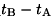and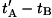can only be the inequality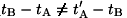and can never be an equality. Therefore, because of the synchronicity in K of spatially coinciding clocks, the only conclusion the observers in K can arrive at is that the only relationship betweenandin k can also only be the inequalityand can never be an equality in k. However, for a light source moving with the rod, although the conditionis obeyed, the inequalityis not possible to be true in k. Indeed, recall from the above that the clocks at A and B in k are synchronous which makes it impossible for the inequalityto be true in k when(synchronous clocks can only exhibit the equality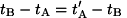when the velocity of light is) On the other hand, for a light source at rest with K the relationship derived in K betweenandis indeed the inequalityand therefore, because of the synchronicity in K of spatially coinciding clocks, the relationship betweenandin k is indeed the inequality. Recall again from the above, however, that the clocks at A and B in k are synchronous, which makes it impossible forto be the velocity of light in k when the true relationship in k, as is in this case, betweenandis the inequality(velocity of light cannot bewhen synchronous clocks exhibit the inequality). Therefore, because of the above-proven persistent synchronicity in all systems of two clocks synchronous in a given system, following from Newton's mechanics valid in K, joint validity of the inequality, which Einstein's theory of relativity always inevitably derives in k and the equalityin k can never be achieved. As seen above, for a light source at rest with k, whereby, the relationship between the time intervalsandread from the faces of synchronous clocks in k must necessarily be the equality, which Einstein's "theory" of relativity can never derive for k. On the contrary, for a light source moving with respect to k synchronous clocks in k can exhibit the inequalityin k if and only if the velocity of light is expressed by the inequality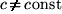. It may be more convenient for some to understand the untenability of the second postulate by following the links given below, presenting arguments which are essentially variations of the same above-discussed arguments: The second postulate leads to contradictory statements about the synchronicity of two clocks in the same system (moving system k). The formula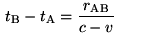(respectively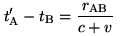) from §2 of Einstein's 1905 paper violates directly the second postulate. One should note also that the failure of Einstein's "theory" of relativity is only indirectly connected with the non-physicality of the Lorentz transformations. Einstein's theory of relativity cannot derive these transformations despite the impression created that it does. Also, the Lorentz transformations are by no means synonymous with Einstein's theory of relativity as is often presented to be the case. It should be noted that unlike Einstein's theory of relativity, no parts of which make any sense, Lorentz transformations are perfectly mathematically consistent. The problem is that they lack physical meaning, which can be proved by arguments only tangentially similar to the arguments invalidating Einstein's theory of relativity. Further, as can be seen at once, Einstein's theory of relativity, specifically its first postulate, is in an unresolvable conflict with the Lorentz transformations. As a detail, the latter means that Einstein's theory of relativity cannot derive the mass-energy relationship, popularly known as E = mc2, despite the vigorous propaganda insisting that it is Einstein's theory of relativity that has derived that mass-energy relationship. References 1. Einstein A., The Principle of Relativity, Dover, 37-65, 1952 - English translation of the original: Einstein A., Annalen der Physik, 17, 891-921 (1905).
 Sources   1905 Paper   1905 (English) Michelson Michelson-Morley Derivations   tB-tA   t'A-tB e-mail